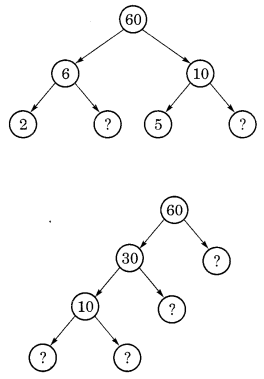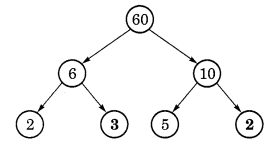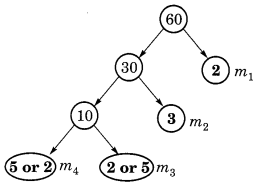## Ex 3.5 Question 1.

Which of the following statements are true?
(a) If a number is divisible by 3, it must be divisible by 9.
(b) If a number is divisible by 9, it must be divisible by 3.
(c) A number is divisible by 18, if it is divisible by both 3 and 6.
(d) If a number is divisible by 9 and 10 both, then it must be divisible by 90.
(e) If two numbers are co-primes, at least one of them must be prime.
(f) All numbers which are divisible by 4 must also be divisible by 8.
(g) All numbers which are divisible by 8 must also be divisible by 4.
(h) If a number exactly divides two numbers separately,- it must exactly divide their sum.
(i) If a number exactly divides the sum of two numbers, it must exactly divide the two numbers separately.
Solution:
(a) False
(b) True
(c) False
(d) True
(e) False
(f) False
(g) True
(h) True
(i) False

## Ex 3.4 Question 2.

Here are two different factor trees for 60. Write the missing numbers.Solution:
(a)(b)## Ex 3.4  Question 3.

Which factors are not included in the prime factorization of a composite number?
Solution:
1 is the factor that is not included in the prime factorization of a composite number.

## Ex 3.4 Question 4.

Write the greatest 4-digit number and express it in terms of its prime factors.
Solution:
The greatest 4-digit number = 9999

Hence, the prime factors of 9999 = 3 x 3 x 11 x 101.

## Ex 3.4 Question 5.

Write the smallest 5-digit number and express it in the form of its prime factors.
Solution:
The smallest 5-digit number = 10000

Hence, the required prime factors: 10000 = 2 x 2 x 2 x 2 x 5 x 5 x 5 x 5.

## Ex 3.4 Question 6.

Find all the prime factors of 1729 and arrange them in ascending order. Now state the relations, if any, between the two consecutive prime factors.
Solution:
Hence, the prime factors of 1729 = 7 x 13 x 19.
Here, 13 – 7 = 6 and 19 – 13 = 6
The difference between two consecutive prime factors is 6.

## Ex 3.4  Question 7.

The product of three consecutive numbers is always divisible by 6. Verify this statement with the help of some examples.
Solution:
Example 1:
Take three consecutive numbers 2, 3 and 4.
2 x 3 x 4 = 24

Therefore, the product 2 x 3 x 4 = 24 is divisible by 6.

Example 2:
Take three consecutive numbers 4 ,5 and 6.
4 x 5 x 6 = 120
Therefore, the product 4 x 5 x 6 = 120 is divisible by 6.

## Ex 3.4 Question 8.

The sum of two consecutive odd numbers is divisible by 4. Verify this statement with the help of some examples.
Solution:
Example 1:
5 + 3 = 8 and 8 is divisible by 4.

Example 2:
7 + 5 = 12 and 12 is divisible by 4.

Example 3:
9 + 7 = 16 and 16 is divisible by 4.

## Ex 3.4 Question 9.

In which of the following expressions, prime factorisation has been done?
(a) 24 = 2 x 3 x 4
(b) 56 = 7 x 2 x 2 x 2
(c) 70 = 2 x 5 x 7
(d) 54 = 2 x 3 x 9.
Solution:
(a) 24 = 2 x 3 x 4
Here, 4 is not a prime number.
Hence, 24 = 2 x 3 x 4 is not a prime factorisation.

(b) 56 = 7 x 2 x 2 x 2
Here, all factors are prime numbers
Hence, 56 = 7 x 2 x 2 x 2 is a prime factorisation.

(c) 70 = 2 x 5 x 7
Here, all factors are prime numbers.
Hence, 70 = 2 x 5 x 7 is a prime factorisation.

(d) 54 = 2 x 3 x 9
Here, 9 is not a prime number.
Hence, 54 = 2 x 3 x 9 is not a prime factorisation.

## Ex 3.4  Question 10.

Determine if 25110 is divisible by 45.
Solution:
45 = 5 x 9
Here, 5 and 9 are co-prime numbers.
Test of divisibility by 5: a unit place of the given number 25110 is 0. So, it is divisible by 5.
Test of divisibility by 9:
Sum of the digits = 2 + 5 + l + l + 0 = 9 which is divisible by 9.
So, the given number is divisible by 5 and 9 both. Hence, the number 25110 is divisible by 45.

## Ex 3.4 Question 11.

18 is divisible by both 2 and 3. It is also divisible by 2 x 3 = 6. Similarly, a number is divisible by both 4 and 6. Can we say that the number must also be divisible by 4 x 6 = 24? If not, give an example to justify your answer.
Solution:
The given two numbers are not co-prime. So, it is not necessary that a number divisible by both 4 and 6, must also be divisible by their product 4 x 6 = 24.

## Ex 3.4 Question 12.

I am the smallest number, having four different prime factors. Can you find me?
Solution:
The smallest 4 prime numbers =  2, 3, 5, and 7.
Hence, the required number = 2 x 3 x 5 x 7 = 210

error: Content is protected !!